Previous Year Questions with Solutions: Acids, Bases & Salts - 1

# Previous Year Questions with Solutions: Acids, Bases & Salts - 1 - Science Class 10

## Very Short Answer Type Questions

Q.1. State the purpose for which litmus is used in laboratories. [2021C]
Ans.
Litmus is used in laboratories as an indicator to determine whether a substance is acidic or basic. It changes color in the presence of acids or bases, with blue indicating a basic solution and red indicating an acidic solution.

Q.1. A student took a small amount of copper oxide in a conical flask and added dilute hydrochloric acid to it with constant stirring. He observed a change in colour of the solution.
(i) Write the name of the compound formed and its colour.
(ii) Write a balanced chemical equation for the reaction involved.      

Ans. (i) The compound formed is copper chloride (CuCl2) and its colour is green in aqueous solution.
(ii) The balanced chemical equation for the reaction is: CuO + 2HCl → CuCl2 + H2O

Q.2. (a) Write a balanced equation to show the reaction that occurs when a piece of aluminium is dipped in a dilute solution of (i) sulphuric acid and (ii) sodium hydroxide.
(b) Write the colour of the solution formed when copper oxide is treated with hydrochloric acid. Give a reason for this observation.      

Ans. (a) (i) 2AI + 3H2SO4 → AI2(SO4)3 + 3H2
(ii) 2AI + 2NaOH + 2H2O → 2NaAIO2 + 3H2
(b) The colour of the solution formed when copper oxide is treated with hydrochloric acid is a bluish-green colour. This is because copper oxide reacts with hydrochloric acid to produce copper chloride and water. The copper chloride formed dissolves in water, resulting in the bluish-green colour of the solution.

Q.3. (i) Suggest a safe procedure for diluting a strong concentrated acid.
(ii) Name the salt formed when sulphuric acid is added to sodium hydroxide and write its pH.
(iii) Dry hydrochloric acid (HCl) gas does not change the colour of dry blue litmus paper. Why?      

Ans. (i) During the dilution of a strong concentrated acid, always add acid to water and not the water to acid. The dissociation of an acid in water is a highly exothermic process, as the acid has a strong affinity for water. Adding water to acid can cause a violent reaction due to the rapid generation of heat. By adding acid to water slowly and with constant stirring, the heat generated can be dissipated more effectively, ensuring a safe dilution process.
(ii) The salt formed when sulphuric acid is added to sodium hydroxide is sodium sulphate (Na2SO4). Sodium sulphate is a neutral salt, and its aqueous solution will have a pH of 7.
(iii) Dry hydrochloric acid (HCl) gas does not change the colour of dry blue litmus paper because the colour change in blue litmus paper is only observed in the presence of hydrogen ions (H+) or hydronium ions (H3O+). HCl can produce these ions only in aqueous solution, where it dissociates to release H+ ions. In the absence of water, HCl gas does not produce H+ ions and, therefore, does not cause a colour change in dry blue litmus paper.

Q.4. Two solutions M and N give red and blue colour respectively with a universal indicator.
(i) In which solution will the hydrogen ion concentration be more? Justify your answer.
(ii) If both M and N solutions are mixed and the resultant mixture is tested with a universal indicator, it turns green. What is the nature of the salt formed? Justify your answer.      

Ans. (i) Solution M gives a red color with a universal indicator, indicating that it is an acidic solution with a higher hydrogen ion concentration. Solution N gives a blue color with a universal indicator, indicating that it is a basic solution with a lower hydrogen ion concentration.
(ii) When solutions M and N are mixed, the resulting green color with a universal indicator indicates that the mixture is neutral. This suggests that a salt solution is formed, which is neither acidic nor basic.

Q.5. On heating X at 373 K, it loses water molecules and becomes Y. Y is a substance which doctors use for supporting fractured bones in the right position.
(i) Identify X and V.
(ii) How can X be reobtained from Y?      
Ans.
(i) X is gypsum (CaSO4 · 2H2O) and Y is Plaster of Paris (CaSO4 · 0.5H2O). V is the substance doctors use for supporting fractured bones in the right position.
(ii) X can be reobtained from V by adding water to Plaster of Paris, which will convert it back to gypsum. The reaction is as follows:
CaSO4 · 0.5H2O + 1.5H2O → CaSO4 · 2H2O

Q.6. Consider the following salts:
(i) yCI
(ii) NH4X
(iii) ZCO3
(a) What would be the pH of the salt solution if in yCI, y is sodium? Give a reason for your answer.
(b) If in salt NH4X, X is nitrate, then its solution will give what colour with a universal indicator? Why?
(c) What would be the change in colour in a blue litmus solution if ZCO3 is added to it and Z is potassium?

Ans. (a) If in yCI, y is sodium, the salt formed is NaCI. NaCI is a salt of a strong acid (HCI) and a strong base (NaOH), making it a neutral salt. Hence, the pH of the salt solution would be 7.
(b) If in salt NH4X, X is nitrate, the salt formed is NH4NO3. NH4NO3 is a salt of a weak base (NH4OH) and a strong acid (HNO3). It is an acidic salt and will give an orange-yellow color with a universal indicator.
(c) If ZCO3 is added to a blue litmus solution and Z is potassium, the solution will turn red. This is because ZCO3 is potassium carbonate, which is a basic salt. It will neutralize the acidity of the blue litmus solution, causing it to turn red.

Q.7. The industrial process used for the manufacture of caustic soda involves electrolysis of an aqueous solution of compound 'X'. In this process, two gases Y and Z are liberated. Y is liberated at the cathode and Z, which is liberated at the anode, on treatment with dry slaked lime, forms a compound 'B'. Name X, Y, Z, and B.      
Ans. Compound X is sodium chloride (NaCl).
Gas Y liberated at the cathode is hydrogen gas (H2).
Gas Z liberated at the anode is chlorine gas (Cl2).
When chlorine gas (Z) is treated with dry slaked lime (Ca(OH)2), it forms bleaching powder (CaOCl2 or B).

Q.8. Out of the two hydrochloric acid and acetic acid, which one is considered as strong acid and why ? Write the name/molecular formula of one more strong acid.       [2021 C]
Ans. Hydrochloric acid is a stronger acid than acetic acid because it dissociate completely in aqueous solution to give H+ ions. H2SO4 (sulphuric acid) is an example of strong acid.

Q.9. (i) Draw a labelled diagram to show the preparation of hydrogen chloride gas in the laboratory.
(ii) Test the gas evolved first with dry and then with wet litmus paper. In which of the two cases does the litmus paper show a change in color?
(iii) State the reason for the exhibiting acidic character by dry HCl gas/HCl solution.      

Ans.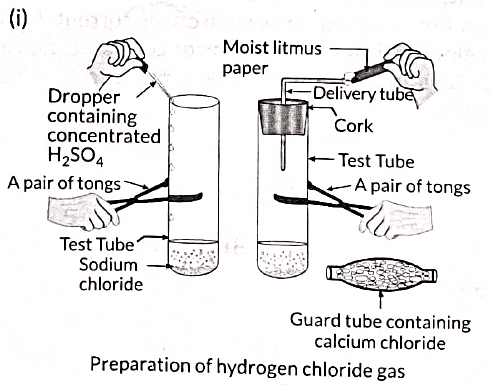(ii) The litmus paper does not show any change in color when tested with dry blue litmus paper. However, when tested with moist blue litmus paper, it turns red. This indicates that hydrogen chloride (HCl) gas does not exhibit acidic behavior in the absence of water but shows acidic behavior in the presence of water.
(iii) When hydrogen chloride (HCl) gas dissolves in water, it forms hydrochloric acid solution (HCl(aq)). In the solution, HCl dissociates and produces H+ or H3O+ ions. The presence of these ions makes HCl solution acidic. The reaction can be represented as: HCl(g) + H2O(l) → H3O+(aq) + Cl-(aq)

Q.10. Complete and balance the following chemical equations:       
(i) NaOH(aq) + Zn(s) →
(ii) CaCO3(s) + H2O(l) + CO2(g) →
(iii) HCl(aq) + H2O(l) →
Ans. (i) 2NaOH(aq) + Zn(s) → Na2ZnO2(aq) + H2(g)
(ii) CaCO3(s) + H2O(l) + CO2(g) → Ca(HCO3)2(aq)
(iii) HCl(aq) + H2O(l) → H3O+(aq) + Cl-(aq)

Q.11. (a) You are provided with concentrated sulphuric acid. Describe the process of preparing a dilute solution of sulphuric acid.
(b) What is the effect of dilution on (H3O+/OH-) ratio?
(c) If the H3O+ ion concentration is increased in a solution, will the pH increase or decrease? What are the probable colours of pH paper if the pH range is 0-5 to 2-0?       
Ans. (i) Dilution of a concentrated acid is a highly exothermic reaction. To prevent potential hazards, the dilution process should be carried out carefully. The recommended method is to take a beaker with a sufficient amount of water and slowly add the concentrated acid to it while stirring. It is important to add acid into water and not the other way around to reduce the risk of splashing or glass breakage due to localized heating.
(ii) Dilution of an acid or base leads to a decrease in the concentration of ions (H3O+ and OH-) per unit volume. Therefore, the (H3O+/OH-) ratio is also reduced.
(iii) If the concentration of H3O+ ions increases in a solution, the pH will decrease. As for the probable colors of pH paper, pH range 0-5 to 2-0 typically corresponds to acidic conditions. The probable colors of pH paper in this range can be red or orange, indicating acidity.

Q.12. List the important products of the Chlor-alkali process. Write one important use of each.       
Ans. The important products of the Chlor-alkali process are sodium hydroxide, chlorine, and hydrogen.

• Sodium hydroxide (NaOH): It is used in the manufacture of soaps and detergents.
• Chlorine (Cl2): It is used as a germicide and disinfectant for sterilization of drinking water and swimming pools.
• Hydrogen (H2): It is used in the manufacture of ammonia, which is used for the preparation of various fertilizers.

Q.13. How is washing soda prepared from sodium carbonate? Give its chemical equation. State the type of this salt. Name the type of hardness of water which can be removed by it?       
Ans. Washing soda is prepared by recrystallization of sodium carbonate. The chemical equation for its preparation is:
Na2CO3(s) + 10H2O(l) → Na2CO3.10H2O(s)
Washing soda is a basic salt. It is used to remove the permanent hardness of water. It can remove the calcium and magnesium ions that cause temporary hardness of water.

Q.14. Give reasons for the following:
(i) Only one half of water molecule is shown in the formula of plaster of Paris.
(ii) Sodium hydrogen carbonate is used as an antacid.
(iii) On strong heating, blue-colored copper sulfate crystals turn white.

Ans. (i) Only one half of the water molecule is shown in the formula of plaster of Paris (CaSO4.1/2H2O) because one molecule of water is being shared by two molecules of calcium sulfate (CaSO4). So the effective water of crystallization for one CaSO4 unit comes to half a molecule of water.
(ii) Sodium hydrogen carbonate (NaHCO3) is used as an antacid because it is a weak base and can react with the excess acid produced in the stomach due to hyperacidity, neutralizing it and providing relief.
(iii) Blue-colored copper sulfate crystals are hydrated copper sulfate (CuSO4.5H2O). On strong heating, these crystals lose their water of crystallization and turn into anhydrous copper sulfate (CuSO4), which is white in color.

Q.15. During electrolysis of brine, a gas 'G' is liberated at the anode. When this gas 'G' is passed through slaked lime, a compound 'C' is formed, which is used for disinfecting drinking water.
(i) Write the formula of 'G' and 'C'.
(ii) State the chemical equations involved.
(iii) What is the common name of compound 'C'? Give its chemical name.       

Ans: (i) The formula of 'G' is Cl2 and the formula of 'C' is CaOCl2.
(ii) The chemical equations involved are:

• At the anode during electrolysis of brine: 2NaCl(aq) + 2H2O(l) → Cl2(g) + H2(g) + 2NaOH(aq)
• When chlorine gas (G) is passed through slaked lime: Ca(OH)2(s) + Cl2(g) → CaOCl2(s) + H2O(l)

(iii) The common name of compound 'C' is bleaching powder. Its chemical name is calcium hypochlorite.

Q.16. Blue litmus solution is added to two test tubes A and B containing dilute HCl and NaOH solution respectively. In which test tube a colour change will be observed? State the colour change and give its reason.       
Ans.
The colour change will be observed in test tube A containing dilute HCl. The blue litmus paper will turn red in test tube A. This is because HCl is an acid and it turns blue litmus paper red.

Q.17. Out of HCI and CH3COOH, which one is a weak acid and why? Explain with the help of an example.       
Ans. Out of HCI and CH3COOH, CH3COOH is a weak acid because it dissociates partially in the solution. This can be proved with the help of the following example. If 1 M HCI and 1 M CH3COOH are taken in the beaker as shown in the figure, greater deflection is observed in the case of HCI. This shows that more ions are produced by HCI in the solution, which produces more current.

Q.18. (a) What does pH scale measure?
(b) Write its range.
(c) State the significance of highest and lowest values of pH scale.
[2019 C]
Ans. (a) pH scale is used to measure the strength of acids and bases.
(b) The pH value ranges from 1 to 14.
(c) Lowest value of pH scale indicates the highly acidic solution. Highest value of pH scale indicates the highly basic solution.

Q.19. "Sodium hydrogen carbonate is a basic salt". Justify this statement. How is it converted into washing soda?        [AI 2019]
Ans.
Sodium hydrogen carbonate (NaHCO3) is basic in nature as on hydrolysis it gives a mixture of strong base (NaOH) and weak acid (H2CO3). Sodium hydrogen carbonate is converted to washing soda in the following way:
(i) Thermal decomposition of NaHCO3:
2 NaHCO3(s) → Na2CO3(s) + CO2(g) + H2O(g)
(ii) Recrystallization of sodium carbonate:
Na2CO3 + 10H2O → Na2CO3·10H2O (solid)
Thus, sodium hydrogen carbonate is converted into washing soda through thermal decomposition and recrystallization.

Q.20. A solution 'X' gives orange colour when a drop of it falls on pH paper, while another solution Y gives bluish colour when a drop of it falls on pH paper. What is the nature of both the solutions? Determine the pH of solutions 'X' and 'Y'.        

Ans. Solution 'X' is an acidic solution with a pH range of 3-4. Solution 'Y' is a basic solution with a pH of 9.

Q.21. On adding a few drops of universal indicator in three colorless solutions X, Y, and Z taken separately in three test tubes, a student observed the changes in color as green in X, red in Y, and blue in Z.
(a) Arrange X, Y, and Z in increasing order of their pH values.
(b) Which one of the three X, Y, and Z will change the color of phenolphthalein? Why?        [2019 C]
Ans.
(a) The increasing order of pH values for solutions X, Y, and Z is Y < X < Z.
(b) Solution Z will change the color of phenolphthalein because it is a basic solution that changes the color of phenolphthalein from colorless to pink.

Q.22. Why is sodium hydrogen carbonate an alkaline salt? List its two important uses.        [2019 C]
Ans.
Sodium hydrogen carbonate (NaHCO3) is an alkaline salt because its pH value is greater than 7. It is a salt of a strong base and a weak acid. Two important uses of sodium hydrogen carbonate are:
(i) It is used as an antacid in medicines.
(ii) It is used as an additive in food and drinks.

Q.23. Identify the acid and the base from which sodium chloride is obtained. Which type of salt is it? When is it called rock salt? How is rock salt formed?       
Ans. Sodium chloride is obtained by the neutralization of sodium hydroxide (base) with hydrochloric acid (acid). It is a neutral salt. When sodium chloride is found in the form of solid deposits with impurities, it is called rock salt. Rock salt is formed by the evaporation of salty water from inland lakes.

Q.24. A white powder is added while baking cakes to make it soft and spongy. Name its main ingredients and explain the function of each ingredient. Write the chemical reaction taking place when the powder is heated during baking.       
Ans.
The white powder added while baking cakes to make them soft and spongy is baking powder. Its main ingredients are sodium hydrogen carbonate and a mild edible acid like tartaric acid or citric acid. Sodium hydrogen carbonate decomposes to give out carbon dioxide, which causes the cake to rise and makes it soft and spongy. The function of tartaric acid or citric acid is to neutralize sodium carbonate formed during heating, which can otherwise make the cake bitter. The reaction taking place when the powder is heated is: 2 NaHCO3 (heat) → Na2CO+ H2O + CO2

Q.25. (a) Why is electrolysis of brine called 'Chlor-alkali process'? Write the chemical equation involved in this process.
(b) A few crystals of hydrated copper sulphate are heated in a dry test-tube. Enlist any two observations.       
Ans.
(a) The electrolysis of brine is called the 'Chlor-alkali process' because it produces chlorine (chlor) and sodium hydroxide (alkali). The chemical equation involved in this process is: 2NaCl + 2H2O → 2NaOH + Cl2 + H2
(b) Two observations when hydrated copper sulphate crystals are heated in a dry test-tube are:
(i) The color of copper sulphate crystals becomes white after heating.
(ii) Water droplets are noticed at the mouth side of the boiling tube, which are obtained from the water of crystallization.

Q.26. 2 mL of sodium hydroxide solution is added to a few pieces of granulated zinc metal taken in a test tube. When the contents are warmed, a gas evolves which is bubbled through a soap solution before testing. Write the equation of the chemical reaction involved and the test to detect the gas. Name the gas which will be evolved when the same metal reacts with a dilute solution of a strong acid.       
Ans. It is observed that active metals like zinc react with strong bases like sodium hydroxide (NaOH) to liberate hydrogen gas (H2) and the corresponding salt. The equation for the chemical reaction is:
2NaOH(aq) + Zn(s) → Na2ZnO2(aq) + H2(g)
The evolution of gas is confirmed by the bubble formation in soap solution. The test to detect hydrogen gas (H2) is as follows: When a burning matchstick is kept on the mouth of the test tube, a "pop" sound is heard, which confirms the presence of hydrogen gas.
When the same metal (zinc) reacts with a dilute solution of a strong acid, hydrogen gas (H2) will also be evolved.

Q.27. The pH of a salt used to make tasty and crispy pakoras is 14. Identify the salt and write a chemical equation for its formation.       
Ans. The salt used to make tasty and crispy pakoras is sodium bicarbonate (NaHCO3). On a large scale, sodium bicarbonate is prepared as follows:
NaCl + H2O + CO2 + NH3 → NH4Cl + NaHCO3
Two uses of sodium bicarbonate are as follows:
(i) It is used as an antacid in medicines.
(ii) It is used as an additive in food and drinks.

Q.28. With the help of an example, explain what happens when a base reacts with a non-metallic oxide. What do you infer about the nature of non-metal oxide?        [Board Term I, 2017]
Ans. When a base reacts with a non-metallic oxide, it forms a salt and water. For example, when calcium hydroxide (a base) reacts with carbon dioxide (a non-metallic oxide), it produces calcium carbonate (salt) and water. The reaction can be represented as follows:
CO2 + Ca(OH)2 → CaCO3 + H2O
From this reaction, we can infer that non-metallic oxides are generally acidic in nature.

Q.29. How will the following substances dissociate to produce ions in their solutions?        [Board Term I, 2017]
(i) Hydrochloric acid
(ii) Sulphuric acid
(iii) Potassium hydroxide
(iv) Magnesium hydroxide
(v) Nitric acid
(vi) Sodium hydroxide
Ans.
The dissociation of the given substances to produce ions in their solutions are as follows:
(i) Hydrochloric acid (HCI): HCI → H+ + Cl-
(ii) Nitric acid (HNO3): HNO3 → H+ + NO3-
(iii) Sulphuric acid (H2SO4): H2SO4 → 2H+ + SO42-
(iv) Sodium hydroxide (NaOH): NaOH → Na+ + OH-
(v) Potassium hydroxide (KOH): KOH → K+ + OH-
(vi) Magnesium hydroxide [Mg(OH)2]: Mg(OH)2 → Mg2+ + 2OH-

Q.30. Explain how an antacid works.       [Board Term 1, 2017]
Ans. An antacid works by neutralizing excess stomach acid, which can cause indigestion, pain, and irritation. It contains a base, such as magnesium hydroxide (Milk of Magnesia), which reacts with the excess hydrochloric acid in the stomach to form a salt and water. The reaction can be represented as follows:
Mg(OH)2 + 2HCl → MgCl2 + 2H2O
The formed salt, magnesium chloride, is neutral and helps alleviate the symptoms of indigestion.

Q.31. Answer the following questions:     [CBSE 2016]
(i) State the colour of phenolphthalein in soap solution.
(ii) Name the by-product of chlor-alkali process which is used for the manufacture of bleaching powder.
(iii) Name one indicator which specifies the Various levels of H+ ion concentration.
Ans.

(i) Pink
(ii) Chlorine
(iii) Universal indicator

Q.32. On passing excess carbon dioxide gas through lime water, it first turns milky and then becomes colourless. Explain why? Write all the chemical equations of the reactions involved.    [CBSE 2016]
Ans.
Ca(OH)2 + CO2 → CaCO3(s) + H2O
CaCO3(s) + CO2(g) + H2O(l) → Ca(HCO3)2
Lime water turns milky due to the formation of calcium carbonate. It becomes colourless when excess of CO2 is passed due to formation of Ca(HCO3)2 which is soluble in water.

Q.33. Name of type of chemical reaction represented by the following equation :    [CBSE 2016]
(i) CaO + H2O → Ca(OH)2
(ii) 3BaCl2 + Al2 (SO4)3 → 2AlCl3 + 3BaSO4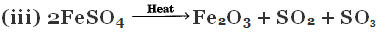Ans.
(i) Combination reaction.
(ii) Double displacement reaction.
(iii) Decomposition reaction.

Q.34.
(a) Define olfactory indicators. Name two substances which can be used as olfactory indicators.
(b) Choose strong acids from the following :
CH3COOH, H2SO4, H2CO3, HNO3     [CBSE 2016]
Ans.

(a) Indicators which work on the basis of odour are called olfactory indicators.
Onion and vanilla extracts can be used as olfactory indicators.
(b) H2SO4 and HNO3 are strong acids out of the four acids given.

Q.35.
(a) A student detected the pH of four unknown solutions A, B, C and D as follows: 11, 5, 7 and 2.
Predict the nature of the solution.
(b) Explain how an antacid works.    [CBSE 2015]
Ans.
(a) A is basic, ‘B’ is acidic ‘C’ is neutral and ‘D’ is strongly acidic.
(b) Hyperacidity is Caused by excess of hydrochloric acid in stomach. Antacid is basic in nature. It neutralises excess of acid and releases CO2 gas which gives relief from pain caused by hyperacidity.

Q.36. Explain the action of dilute hydrochloric acid on the following with chemical equations:    [CBSE 2015]
(a) Magnesium ribbon
(b) Sodium hydroxide
(c) Crushed egg shells
Ans.
(a) Hydrogen gas will be formed, e.g.
Mg (s) + 2HCl (dil) → MgCl2 (aq) + H2 (s)
(b) Sodium chloride and water will be formed, e.g.
NaOH + HCl → NaCl + H2O (Neutralization reaction)
(c) Crushed egg shells are made up of CaCO3 which reacts with dil HCl to give brisk effervescence due to CO2, e.g.
CaCO3 (s) + 2HCl → CaCl2 + H2O + CO2

Q.37. What is meant by water of crystallisation? How would you show that copper sulphate crystals contain water of crystallisation? [CBSE 2015]
Ans. Water which is present in the crystals of a compound is called water of crystallisation. It is defined as the fixed number of water molecules present in one formula unit of a salt. Copper sulphate crystals are blue in colour. On heating solid copper sulphate crystals, they lose water of crystallisation and become white. On adding a few drops of water to the white powder, blue colour of copper sulphate is restored. This shows that copper sulphate crystals contain water of crystallisation.

Q.38. Define water of crystallisation with two examples. How will you prove their existence in the examples given by you? [CBSE 2015]
Ans. Number of fixed water molecules associated with one formula unit of a substance is called water of crystallisation.
Example.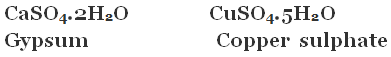(i) Gypsum on heating loses half molecule of water and forms plaster of Paris.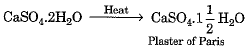On adding water, it again converts to gypsum.
(ii) Copper sulphate on heating loses water molecules and is converted to white anhydrous CuSO4. On adding water to anhydrous copper sulphate, the blue colour of copper sulphate is restored.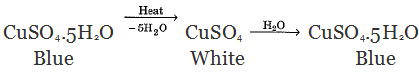Q.39.
(a) Three acidic solutions A, B and C have p H = 0, 3 and 5 respectively.
(i) Which solution has highest concentration of H+ ions?
(ii) Which solution has the lowest concentration of H+ ions?
(b) How concentrated sulphuric acid can be diluted? Describe the process.    [CBSE 2015]
Ans.

(a)
(i) Solution A has the highest concentration of H+ ions.
(ii) Solution C has the lowest concentration of H+ ions.
(b) While diluting concentrated sulphuric acid, water is not added to the acid, rather acid is added to water.
Take some water in a beaker. Add cone, sulphuric acid in small lots to water. Stir the mixture with glass rod constantly. Cool the beaker under water if it becomes hot.

Q.40. Give the chemical name and formula of bleaching powder. What happens when it is exposed to air? Mention two uses of bleaching powder.    [CBSE 2015]
Ans.
Chemical name of bleaching powder is calcium oxychloride.
Formula: CaOCl2 CaOCl2 On exposure to air, it absorbs moisture.
Uses:
(i) As an oxidising agent in chemical industries.
(ii) For disinfecting drinking water to make it free from germs.

Q.1. A cloth strip dipped in onion juice is used for testing a liquid 'X'. The liquid 'X' changes its odour. Which type of an indicator is onion juice? The liquid 'X' turns blue litmus red. List the observations the liquid 'X’ will show on reacting with the following:
(a) Zinc granules
(b) Solid sodium carbonate.
Write the chemical equations for the reactions involved.      
Ans. Onion juice is an of factory indicator. Olfactory indicators give one type of odour in acidic medium and a different odour in basic medium. As the liquid 'X' turns blue litmus red, hence it is an acidic solution.
(a) Acids react with active metals such as zinc, magnesium, etc. and evolve hydrogen gas. The observation with zinc granules will be the evolution of hydrogen gas. The chemical equation for the reaction is: Zn(s) + dil.H2SO4(aq) → ZnSO4(aq) + H2(g)
(b) Acids react with metal carbonates to give carbon dioxide with brisk effervescence. The observation with solid sodium carbonate will be the release of carbon dioxide gas. The chemical equation for the reaction is:
2HCl(aq) + Na2CO3(s) → 2NaCl(aq) + H2O(l) + CO2(g)

Q.2. A chemical compound 'X' is used in the soap and glass industry. It is prepared from brine.
(a) Write the chemical name, common name and chemical formula of 'X'.
(b) Write the equation involved in its preparation.
(c) What happens when it is treated with water containing Ca or Mg salts?      
Ans. (a) The chemical name of 'X' is Sodium Carbonate, commonly known as washing soda. Its chemical formula is Na2CO3.
(b) The equation involved in the preparation of Sodium Carbonate is:
2NaCl + H2O + CO2 → Na2CO3 + 2HCl
(c) When Sodium Carbonate is treated with water containing calcium (Ca) or magnesium (Mg) salts, it forms insoluble precipitates. For example:
Na2CO3 + CaCl2 → CaCO3 + 2NaCl
Na2CO3+ MgSO4 → MgCO3 + Na2SO4

Q.3. (a) The soil in a field is highly acidic. List any two materials which can be added to this soil to reduce its acidity. Give the reason for your choice. [CBSE 2016]
(b) A gas produced in the laboratory is highly soluble in water. Its colourless solution turns pink when a few drops of phenolphthalein is added to it. What is the nature of this gas?
Ans.

(a)
(i) Lime, (CaO) can be added to neutralize acidity.
(ii) Chalk, (CaCO3) can also be added to neutralise acidity.
It is because CaO and CaCO3 are basic in nature which neutralize acid present in soil.
(b) The nature of gas is basic because it turns phenolphthalein pink. The following reaction takes place in aqueous solution,
Example:  NH3 + H2O → NH4+ + OH-

Q.4.
(a) The pH of soil A is 7.5 while that of soil B is 4.5. Which of the two soils A or B should be treated with powdered chalk to adjust its pH and why?    [CBSE 2016]
(b) Name the chemical which is injected into the skin of a person:
(i) During an ant’s sting
(ii) During the nettle leaf sting.
How can the effect of these stings be neutralised?
(c) Explain how the pH change in the river water can endanger the lives of aquatic animals like fish?
Ans.

(a) Soil ‘B’ is acidic. It should be treated with powdered chalk which is basic so as to adjust its pH.
(b)
(i) Formic acid, (HCOOH).
(ii) Formic acid, (HCOOH).
The effect of these stings neutralized by rubbing the skin with mild base like baking soda (NaHCO3).
(c) If pH of river water changes, amount of oxygen dissolved in water may decrease. Acidic and basic water is harmful for skin of aquatic animals like fish.

Q.5. State reasons for the following statements:
(i) Stain of curry on a white cloth becomes reddish brown when soap is scrubbed on it and turns yellow again when the cloth in washed with plenty of water.
(ii) Curd should not be kept in copper or brass vessels. What is done to protect it?    [CBSE 2016]
Ans.
(i) Turmeric reacts with sodium hydroxide present in soap to form red coloured compound. It turns yellow again because sodium hydroxide becomes very dilute on adding lot of water and reaction stops.
(ii) Curd contains lactic acid which reacts with copper or brass vessels and taste changes. Curd should be kept in glass, steel or ceramic container which does not react with lactic acid present in it.

Q.6.
(a) What is pH value of salt formed by a
(i) Weak acid and strong base?
(ii) Strong acid and strong base?
(b) 15 mL of water and 10 ml of sulphuric acid are to be mixed in a beaker
(i) State the method that should be followed with reason.
(ii) What is this process called?    [CBSE 2015]
(c) What is observed when sulphur dioxide is passed through
(i) water (ii) lime water?

Also write chemical equations for the reactions that take place.
Ans.
(a)
(i) The pH value will be greater than 7.
(ii) The pH value will be less that than 7.
(b)
(i) The acid is to be added slowly in water to prevent the mixture to be from being splashed. The  reaction is highly exothermic, therefore, constant cooling should be done.
(ii) The process is called dilution.
(c)
(i) Sulphurous acid is formed.
SO2 + H2O → H2SO3 (Sulphurous acid)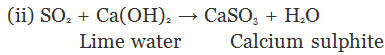Lime water turned turns milky due to formation of calcium sulphite.

Q.7.
(a) State the chemical properties on which the following uses of baking soda are based:
(i) as an antacid
(ii) as soda-acid fire extinguisher
(iii) to make bread and cake soft and spongy.
(b) How washing soda is obtained from baking soda? Write balanced chemical equation.    [CBSE 2015]
Ans.
(a)
(i) It is weakly basic in nature and neutralises hyperacidity.
(ii) It liberates CO2 with H2SO4 reacts with it, which extinguisher fire.
(iii) It liberates CO2 on heating which makes bread and cake soft and sponge.
(b)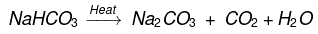Baking soda on heating gives sodium carbonate which on crystallisation from aqueous solution gives washing soda, e.g.
Na2CO3 + 10H2O → Na2CO3.10H2O

Q.8. State reason for the following statements:    [CBSE 2015]
(a) Tap water conducts electricity whereas distilled water does not.
(b) Dry hydrogen chloride gas does not turn blue litmus red whereas dilute hydrochloric acid does.
(c) During summer season, a milk man usually adds a very small amount of baking soda to fresh milk.
(d) For a dilution of acid, acid is added into water and not water into acid.

(e) Ammonia is a base but does not contain hydroxyl group.
Ans.

(a) Tap water contains ions which conduct electricity, distilled water does not contain ions.
(b) Dry HCl does not form ions but HCl gives H+ and Cl-.
(c) Baking soda does not allow milk to change to lactic acid which makes milk sour.
(d) Adding water to acid is highly exothermic and and acid may splash. Therefore, acid is added to water very slowly with continuous stirring.
(e) Ammonia dissolves in water forms OH-. Therefore, it is basic.
NH3 + H2O → NH4+ + OH-

Q.9.
(a) Define universal indicator. Mention its one use.
(b) Solution A gives pink colour when a drop of phenolphthalein indicator is added to it. Solution B gives red colour when a drop of methyl orange is added to it. What type of solutions are A and B and which one of the solutions A and B will have a higher pH value?
(c) Name one salt whose solution has pH more than 7 and one salt whose solution has pH less than 7.    [CBSE 2015]
Ans.
(a) A universal indicator is a mixture of several indicators. It shows different colours at different concentrations of H+ ion concentrations.
(b) Solution A is alkaline in nature.
Solution B is acidic in nature.
Solution A has a higher pH value.
(c) Salts of weak acid and strong base give a solution which have pH more than 7.
i.e. basic salt
Sodium acetate is an example of such salts.
Salts of strong acid and weak base give a solution which has pH less than 7.
i.e. Acidic salt. Ammonium chloride is an example of such salts.

The document Previous Year Questions with Solutions: Acids, Bases & Salts - 1 | Science Class 10 is a part of the Class 10 Course Science Class 10.
All you need of Class 10 at this link: Class 10

## Science Class 10

78 videos|509 docs|153 tests

## FAQs on Previous Year Questions with Solutions: Acids, Bases & Salts - 1 - Science Class 10

 1. What are acids and bases?Ans. Acids and bases are two types of chemical substances with distinct properties. Acids are characterized by their sour taste, ability to turn blue litmus paper red, and ability to donate hydrogen ions (H+) in solution. Bases, on the other hand, have a bitter taste, turn red litmus paper blue, and can accept hydrogen ions or donate hydroxide ions (OH-) in solution.
 2. What is the pH scale?Ans. The pH scale is a measure of the acidity or alkalinity of a solution. It ranges from 0 to 14, with 7 being neutral. A pH value less than 7 indicates acidity, while a pH value greater than 7 indicates alkalinity. The scale is logarithmic, meaning that each unit represents a tenfold difference in acidity or alkalinity. For example, a solution with a pH of 3 is ten times more acidic than a solution with a pH of 4.
 3. How do acids and bases react with metals?Ans. Acids react with metals to produce salt and hydrogen gas. The reaction involves the acid breaking down and releasing hydrogen ions, which then react with the metal to form a salt. For example, when hydrochloric acid (HCl) reacts with zinc (Zn), it produces zinc chloride (ZnCl2) and hydrogen gas (H2). Bases, on the other hand, do not react with metals in the same way.
 4. What are the common uses of acids and bases?Ans. Acids and bases have numerous practical applications in various industries and everyday life. Some common uses of acids include the production of fertilizers, cleaning agents, and food additives. Bases are used in the production of soaps, detergents, and antacids. They are also employed in industries such as agriculture, pharmaceuticals, and water treatment.
 5. How are acids and bases classified?Ans. Acids and bases are classified based on their chemical properties. Acids can be categorized as strong or weak, depending on their ability to donate hydrogen ions. Strong acids completely dissociate in water, while weak acids only partially dissociate. Bases are classified as strong or weak based on their ability to accept hydrogen ions or donate hydroxide ions. Strong bases fully dissociate, while weak bases only partially dissociate.

## Science Class 10

78 videos|509 docs|153 testsExplore Courses for Class 10 examSignup to see your scores go up within 7 days! Learn & Practice with 1000+ FREE Notes, Videos & Tests.
10M+ students study on EduRev
Track your progress, build streaks, highlight & save important lessons and more!
Related Searches

,

,

,

,

,

,

,

,

,

,

,

,

,

,

,

,

,

,

,

,

,

,

,

,

;